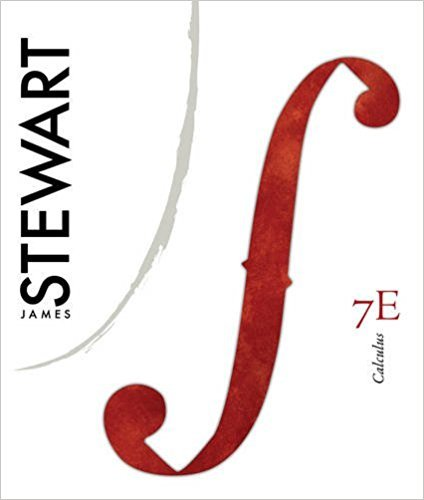×
Get Full Access to Calculus, - 7 Edition - Chapter 14 - Problem 3
Get Full Access to Calculus, - 7 Edition - Chapter 14 - Problem 3

×

# Answer: Sketch the graph of the function.ISBN: 9780538497817 125

## Solution for problem 3 Chapter 14

Calculus, | 7th Edition

• Textbook Solutions
• 2901 Step-by-step solutions solved by professors and subject experts
• Get 24/7 help from StudySoup virtual teaching assistantsCalculus, | 7th Edition

4 5 1 302 Reviews
13
4
Problem 3

Sketch the graph of the function.

Step-by-Step Solution:
Step 1 of 3

Step 2 of 3

Step 3 of 3

##### ISBN: 9780538497817

Calculus, was written by and is associated to the ISBN: 9780538497817. The answer to “Sketch the graph of the function.” is broken down into a number of easy to follow steps, and 6 words. The full step-by-step solution to problem: 3 from chapter: 14 was answered by , our top Calculus solution expert on 11/10/17, 05:23PM. This full solution covers the following key subjects: function, graph, sketch. This expansive textbook survival guide covers 17 chapters, and 887 solutions. This textbook survival guide was created for the textbook: Calculus,, edition: 7. Since the solution to 3 from 14 chapter was answered, more than 271 students have viewed the full step-by-step answer.

Unlock Textbook Solution